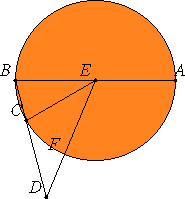Proposition 9

If the side of the hexagon and that of the decagon inscribed in the same circle are added together, then the whole straight line has been cut in extreme and mean ratio, and its greater segment is the side of the hexagon.

Let ABC be a circle, and of the figures inscribed in the circle ABC let BC be the side of a decagon, and CD that of a hexagon, and let them be in a straight line.

I say that the whole straight line BD is cut in extreme and mean ratio, and CD is its greater segment.III.1

Take the center E of the circle, join EB, EC, and ED, and carry BE through to A.

Since BC is the side of an equilateral decagon, therefore the circumference ACB is five times the circumference BC. Therefore the circumference AC is quadruple CB.

VI.33

But the circumference AC is to CB as the angle AEC is to the angle CEB. Therefore the angle AEC is quadruple the angle CEB.

And, since the angle EBC equals the angle ECB, therefore the angle AEC is double the angle ECB.

And, since the straight line EC equals CD, for each of them equals the side of the hexagon inscribed in the circle ABC. Therefore the angle CED also equals the angle CDE. Therefore the angle ECB is double the angle EDC.

But the angle AEC was proved double the angle ECB, therefore the angle AEC is quadruple the angle EDC. And the angle AEC was also proved quadruple the angle BEC, therefore the angle EDC equals the angle BEC.

I.32

But the angle EBD is common to the two triangles BEC and BED, therefore the remaining angle BED equals the remaining angle ECB. Therefore the triangle EBD is equiangular with the triangle EBC.

VI.4

Therefore, proportionally DB is to BE as EB is to BC.

But EB equals CD. Therefore BD is to DC as DC is to CB. And BD is greater than DC, therefore DC is also greater than CB.

Therefore the straight line BD is cut in extreme and mean ratio, and DC is its greater segment.

Therefore, if the side of the hexagon and that of the decagon inscribed in the same circle are added together, then the whole straight line has been cut in extreme and mean ratio, and its greater segment is the side of the hexagon.

Q.E.D.

Guide

Use of this proposition

This result is used in the construction of an icosahedron in propositions XIII.16 and XIII.18.